# Thevenin Equivalent Circuit

• Engineering

## Homework Statement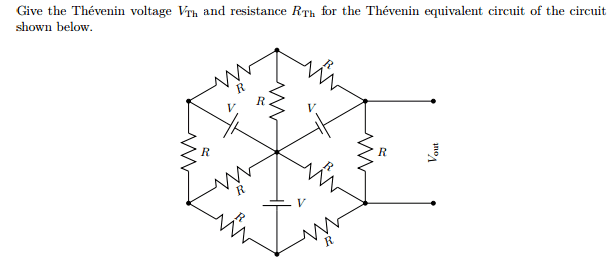## Homework Equations

Thevenin Equivalents
V = IR

## The Attempt at a Solution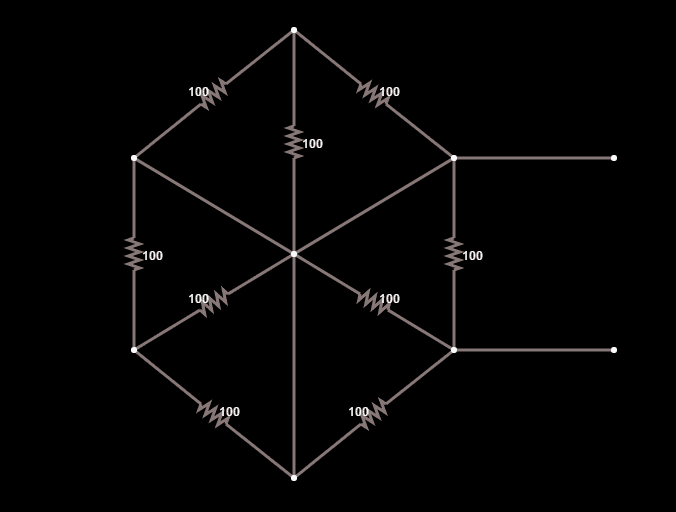To try to find R_th, I replaced all the voltages with short circuits but I do not what to do next.

Then I believe V_th would just be the same as the voltage of the rightmost resistor.

Last edited:

phinds
Gold Member
...I do not know how to combine resistances when there are short circuits.
That implies you do not know how to combine resistances at all. I mean, if you take two series resistance, there is a short circuit (aka "a wire") between the two and it's even worse with parallel resistors since they have short circuits (aka "wires") between pairs of ends.

NascentOxygen
Staff Emeritus
All points joined together by a short-circuit become a single node. So redraw the resistor circuit simplified by condensing nodes along each short-circuit wire into a single node.

All points joined together by a short-circuit become a single node. So redraw the resistor circuit simplified by condensing nodes along each short-circuit wire into a single node.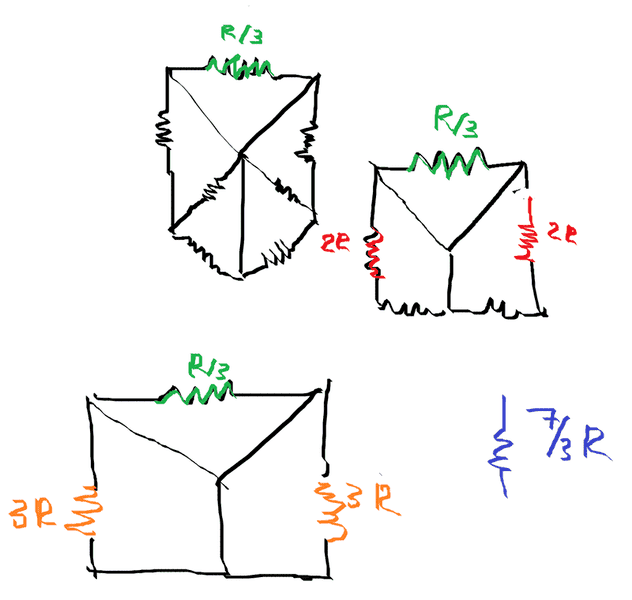This is how I ended up reducing the circuit, was this correct?

NascentOxygen
Staff Emeritus
We are interested only in the resistance seen by the 2 external terminals. Other bits and pieces can be ignored.

Would the resistance just then be 3R?

NascentOxygen
Staff Emeritus
Would the resistance just then be 3R?
No. Where is your simplified circuit showing the external terminals?

A consequence of a resistor having its ends shorted together is that no current will flow in that resistor (there being zero PD across it) so you can omit it from the circuit.

See if this helps.

Starting diagram:
I've replaced resistors with red lines.
The short circuit jumpers are shown in black.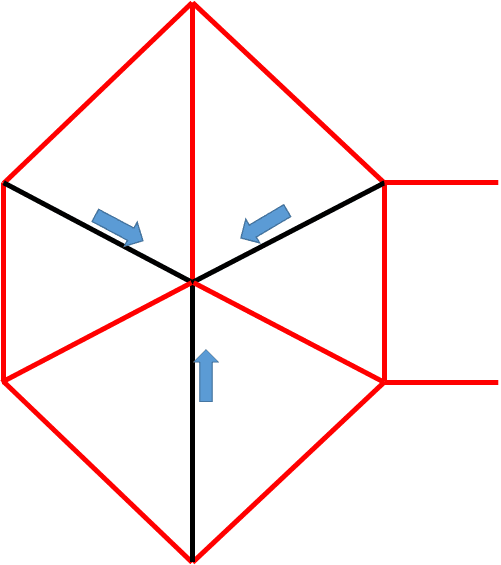The blue arrows show the direction the short circuit jumpers will collapse to the center.

Let's just take the upper left section and see what happens when we collapse its short circuit jumper.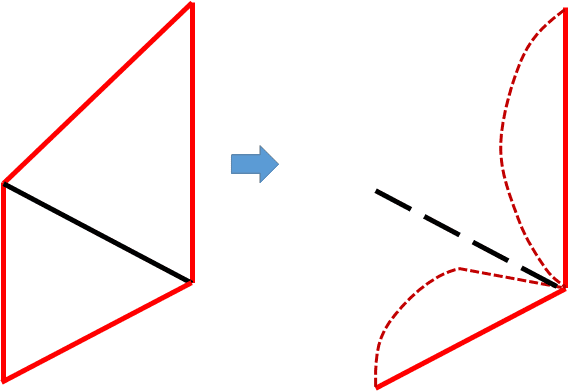Now I have two resistors connected between the outside and the inside of the diagram. The straight red line is the original resistor and the curved red one is the one which moves due to the short circuit jumper.

This leads to an equivalent R/2 between those points.

You need to pursue this with the rest of the circuit and see what you get.

Sorry I didn't have the energy to draw resistors but I hope you get the point.